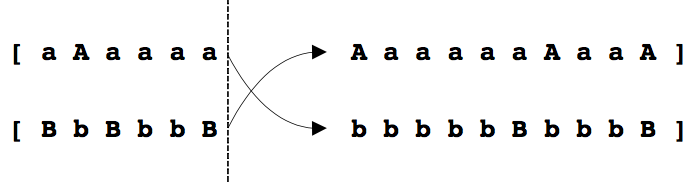## Modeling

My modeling research focuses on thermo-fluids and fundamentals. I am interested in deepened thermodynamic understanding, allowing for new physical interpretations and more efficient models (mixing, combustion, vapor-liquid equilibria). But I am also curious about how unconventional/emerging modeling can be used in mechanical and aerospace engineering (vorticity confinement, deep learning, genetic algorithms).

### Reactive real fluid tabulation

Real fluid computational fluid dynamics require the solution of a real fluid equation of state to calculate temperature and pressure from density and energy. Cubic equations of state, such as Peng-Robinson, are popular because of their computational efficiency and simple generalizability for mixtures. However, their error may be significant (e.g. 20% of the liquid density, or 50% of the transcritical heat capacity). By realizing that real fluid behavior may be isolated to the jet stream in injection problems [article], a new kind of mixing model for reactive real fluid mixtures allows to use tabulated high-accuracy real fluid data for the computational cost of an ideal gas computation [article].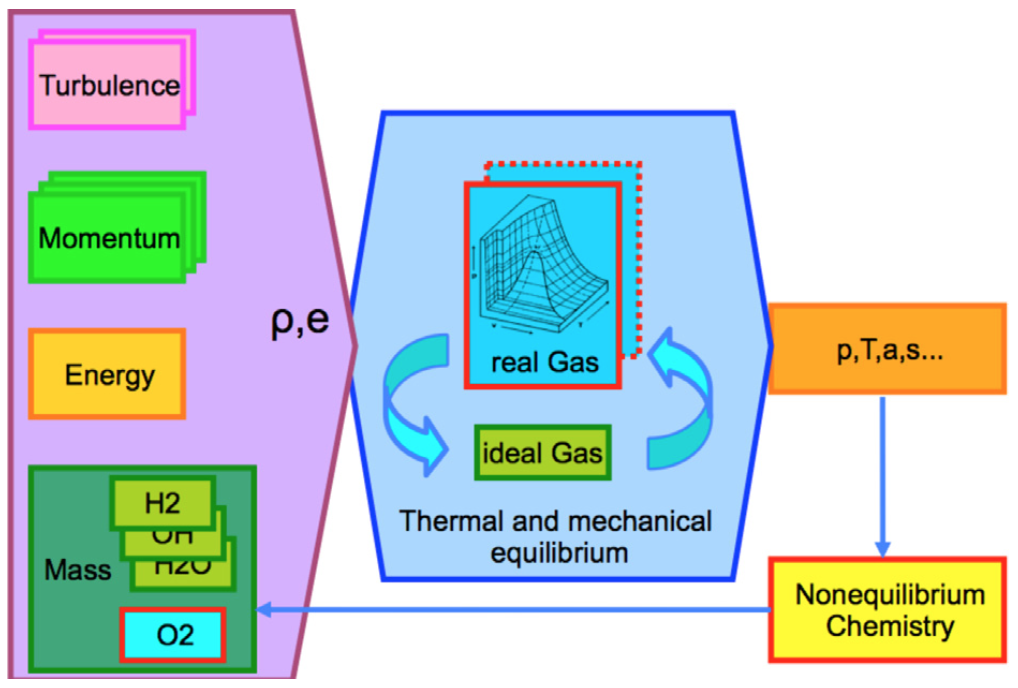### Deep learning flow solver

This is an ongoing project that has now reached the state of a proof-of-concept. The key idea is to use machine learning for a fluid simulation. A deep neural net in an approach similar to convolutional neural nets is used to predict the time-advancement. Time-stepping over several hundred steps qualitatively captures expected flow features; a new approach to evaluate the residuals and training exploiting physical invariants is expected to improve performance.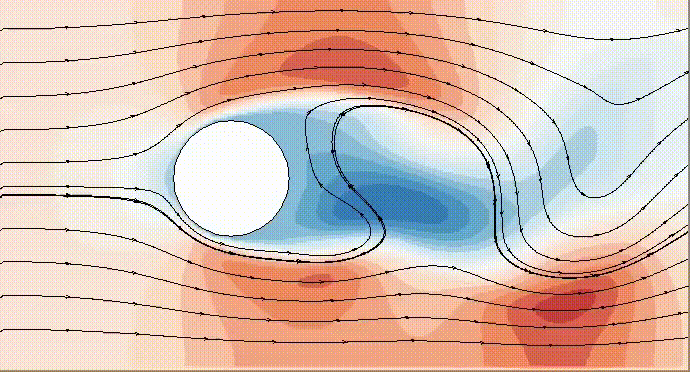### Vorticity confinement

Coarse meshes and low-order discretization allow for fast computation at the cost of high numerical dissipation. This results e.g. in the dissipation of vorticies over unphysically short distances. Vorticity confinement locally detects a vortical layer or filament and injects momentum tangentially to stabilize the vortex, countering the likewise unphysical numerical dissipation. This method is in use mostly in computer graphics but could be useful for engineering applications in rapid prototyping, or to provide initial solutions.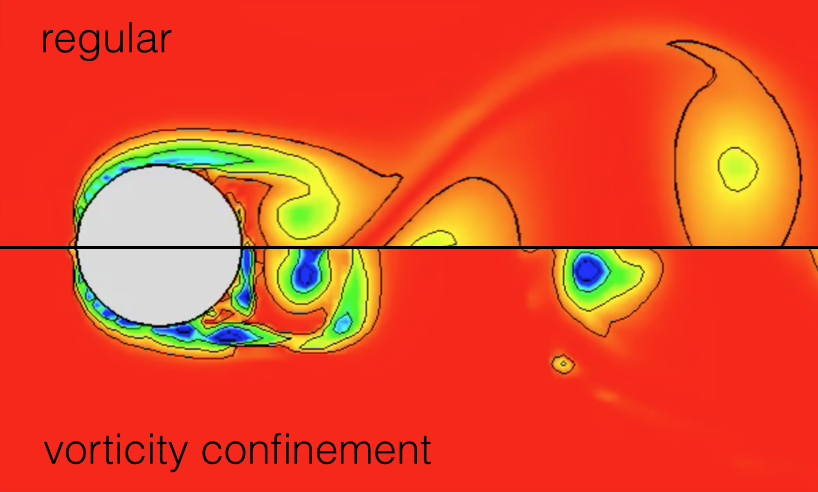### Vapor liquid equilibria in injection simulations

Injection at pressures exceeding the propellant critical pressures is typically considered a diffuse interface mixing process rather than a sharp interface break-up. However, this is not necessarily the case in mixtures where the local mixture critical pressure may exceed the value of the pure components. By splitting the heat and mass transport and the thermodynamic phase calculation, we can determine the equilibrium state on the basis of a large eddy simulation combined with a vapor-liquid equilibrium computation of the mixing process for the three technically relevant mixing problems of inert nitrogen/n-dodecane, inert liquid oxygen/gaseous hydrogen, and reacting liquid oxygen/gaseous hydrogen. The last case represents the first phase analysis of a reacting case. Counterintuitively, the reacting LOX/GH2 shearlayer is more susceptible to phase separation than the inert mixing case, despite the high temperatures reached in the flame. [article].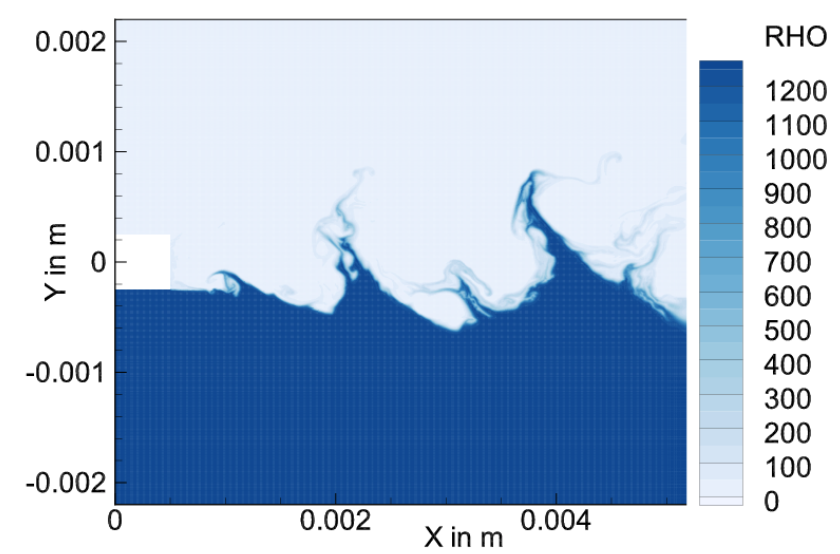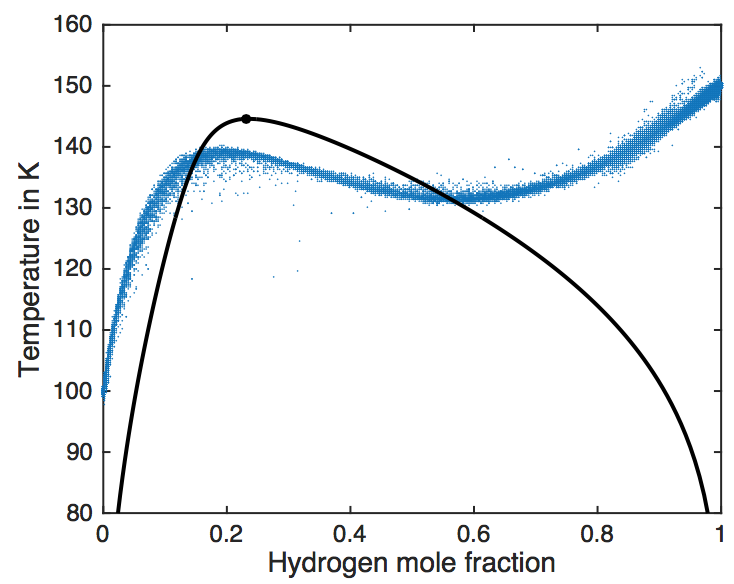### Genetic algorithms in combustion

Genetic algorithms provide a robust approach to optimization. In each generation, a large number of parameter sets are evaluated in terms of a cost function. Successful performance of a set increases its probability of being passed into the next generation, where new parameter sets are created by combination. This approach was successfully used to create models for the the laminar flame velocity of premixed methane-air flames with varying fractions of exhaust gas recirculation for application in large eddy simulations.